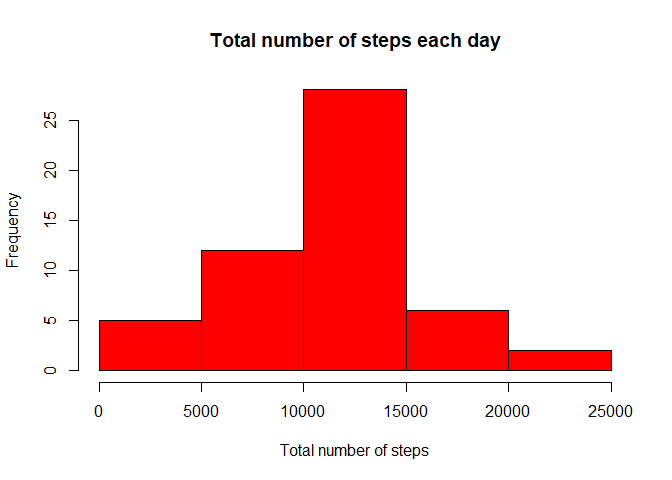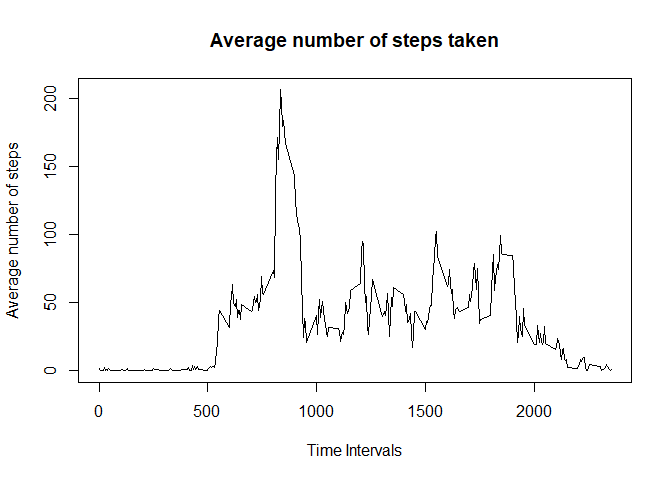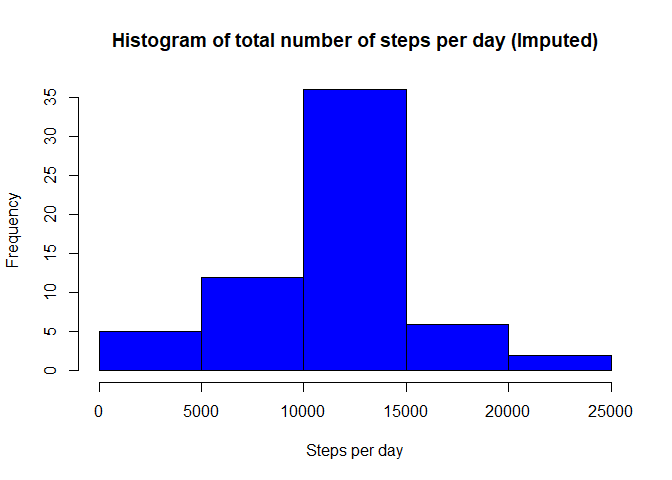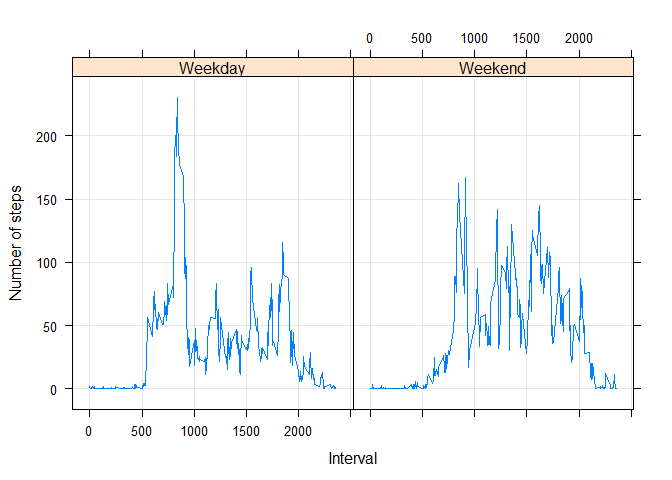# Introduction

It is now possible to collect a large amount of data about personal movement using activity monitoring devices such as a Fitbit, Nike Fuelband, or Jawbone Up. These type of devices are part of the “quantified self” movement – a group of enthusiasts who take measurements about themselves regularly to improve their health, to find patterns in their behavior, or because they are tech geeks. But these data remain under-utilized both because the raw data are hard to obtain and there is a lack of statistical methods and software for processing and interpreting the data.

This assignment makes use of data from a personal activity monitoring device. This device collects data at 5 minute intervals through out the day. The data consists of two months of data from an anonymous individual collected during the months of October and November, 2012 and include the number of steps taken in 5 minute intervals each day.

The data for this assignment can be downloaded from the course web site:

Dataset: Activity monitoring data [52K] The variables included in this dataset are:

steps: Number of steps taking in a 5-minute interval (missing values are coded as NA) date: The date on which the measurement was taken in YYYY-MM-DD format interval: Identifier for the 5-minute interval in which measurement was taken The dataset is stored in a comma-separated-value (CSV) file and there are a total of 17,568 observations in this dataset.

Review criteria less Repo

Valid GitHub URL At least one commit beyond the original fork Valid SHA-1 SHA-1 corresponds to a specific commit Commit containing full submission

1. Code for reading in the dataset and/or processing the data
2. Histogram of the total number of steps taken each day
3. Mean and median number of steps taken each day
4. Time series plot of the average number of steps taken
5. The 5-minute interval that, on average, contains the maximum number of steps
6. Code to describe and show a strategy for imputing missing data
7. Histogram of the total number of steps taken each day after missing values are imputed
8. Panel plot comparing the average number of steps taken per 5-minute interval across weekdays and weekends

### 1. Code for reading in the dataset and/or processing the data

# Running libraies
# Libraries
library(ggplot2)
library(lattice) 
# 1.  Code for reading in the dataset and/or processing the data
actData <- read.csv("./data/activity.csv", as.is = TRUE)
dim(actData)
##  17568     3
    names(actData)
##  "steps"    "date"     "interval"
    head(actData)
##   steps       date interval
## 1    NA 2012-10-01        0
## 2    NA 2012-10-01        5
## 3    NA 2012-10-01       10
## 4    NA 2012-10-01       15
## 5    NA 2012-10-01       20
## 6    NA 2012-10-01       25
    str(actData)
## 'data.frame':    17568 obs. of  3 variables:
##  $steps : int NA NA NA NA NA NA NA NA NA NA ... ##$ date    : chr  "2012-10-01" "2012-10-01" "2012-10-01" "2012-10-01" ...
##  $interval: int 0 5 10 15 20 25 30 35 40 45 ...  sum(is.na(actData$steps))/dim(actData)[]
##  0.1311475

#### Cleaning Data. Data without NAs value

    realAct <- actData[complete.cases(actData), ]
summary(realAct)
##      steps            date              interval
##  Min.   :  0.00   Length:15264       Min.   :   0.0
##  1st Qu.:  0.00   Class :character   1st Qu.: 588.8
##  Median :  0.00   Mode  :character   Median :1177.5
##  Mean   : 37.38                      Mean   :1177.5
##  3rd Qu.: 12.00                      3rd Qu.:1766.2
##  Max.   :806.00                      Max.   :2355.0

### 2. Histogram of the total number of steps taken each day

#  Getting the steps per day by using tapply.
#  The data does not have NAs values.
stepsPerDay <- with(realAct, tapply(steps, date, FUN=sum))
# png("plot1.png")
hist(stepsPerDay,
col="red",
main = "Total number of steps each day",
xlab = "Total number of steps")# dev.off()

### 3. Mean and median number of steps taken each day

    summary(stepsPerDay)
##    Min. 1st Qu.  Median    Mean 3rd Qu.    Max.
##      41    8841   10765   10766   13294   21194
    meanSteps = round(mean(stepsPerDay, na.rm = TRUE))
medianSteps = median(stepsPerDay, na.rm = TRUE)
print (paste("Mean number of steps taken each day is ",
meanSteps,
" and the median number of steps taken each day is  ",
medianSteps
)
)
##  "Mean number of steps taken each day is  10766  and the median number of steps taken each day is   10765"

### 4. Time series plot of the average number of steps taken

    # Average steps per interval.
aggInterval <- aggregate(steps ~ interval, realAct, mean)

# Plot the time series with appropriate labels and heading
plot(aggInterval$interval, aggInterval$steps,
type='l',
col=1,
main="Average number of steps taken",
xlab="Time Intervals",
ylab="Average number of steps"
)### 5. The 5-minute interval that, on average, contains the maximum number of steps

    # Identify the 5-minute interval index.
intIndex <- which.max(aggInterval$steps) # Identify the specific interval and the average steps for that interval print (paste("The interval with the highest average steps is ", aggInterval[intIndex, ]$interval,
".  The maximum number of steps based on that interval is ",
round(aggInterval[intIndex, ]$steps, digits = 1 ) ) ) ##  "The interval with the highest average steps is 835 . The maximum number of steps based on that interval is 206.2" ### 6. Code to describe and show a strategy for imputing missing data  # Calculate the number of rows with missing values. Also done in step #1 dataNA <- actData[!complete.cases(actData), ] nrow(dataNA) ##  2304  # Loop thru all the rows of activity, find the one with NA for steps. # For each identify the interval for that row # Then identify the avg steps for that interval in avg_steps_per_interval # Substitute the NA value with that value ### 7. Histogram of the total number of steps taken each day after missing  # values are imputed for (i in 1:nrow(actData)) { if(is.na(actData$steps[i])) {
val <- aggInterval$steps[which(aggInterval$interval == actData$interval[i])] actData$steps[i] <- val
}
}

# Aggregate the steps per day with the imputed values
imputeSteps <- aggregate(steps ~ date, actData, sum)

# Draw a histogram of the value
# png("plot2.png")
hist(imputeSteps$steps, col="blue", main = "Histogram of total number of steps per day (Imputed)", xlab = "Steps per day")# dev.off() # Compute the mean and median of the imputed value # Calculate the mean and median of the total number of steps taken per day round(mean(imputeSteps$steps))
##  10766
    ##  10766
median(imputeSteps$steps) ##  10766.19  ##  10766.19  ### 8. Panel plot comparing the average number of steps taken per 5-minute  # interval across weekdays and weekends day <- weekdays(as.Date(actData$date))
daylevel <- vector()
for (i in 1:nrow(actData)) {
if (day[i] == "Saturday") {
daylevel[i] <- "Weekend"
} else if (day[i] == "Sunday") {
daylevel[i] <- "Weekend"
} else {
daylevel[i] <- "Weekday"
}
}
actData$daylevel <- daylevel actData$daylevel <- factor(actData\$daylevel)

stepsByDay <- aggregate(steps ~ interval + daylevel, data = actData, mean)
names(stepsByDay) <- c("interval", "daylevel", "steps")

#Make a panel plot containing a time series plot (i.e. type = “l”) of the 5-minute interval (x-axis) and the average number of steps taken, averaged across all weekday days or weekend days (y-axis). The plot should look something like the following, which was creating using simulated data:

library(lattice)
# dev.copy(png, file="plot3.png", height=480, width=480)
xyplot(steps ~ interval | daylevel,
stepsByDay,
type = "l",
grid = TRUE,
layout = c(2, 1),
xlab = "Interval",
ylab = "Number of steps")# dev.off()